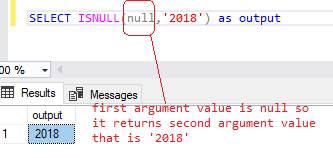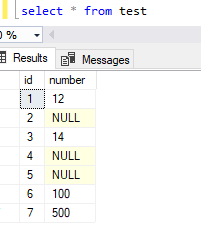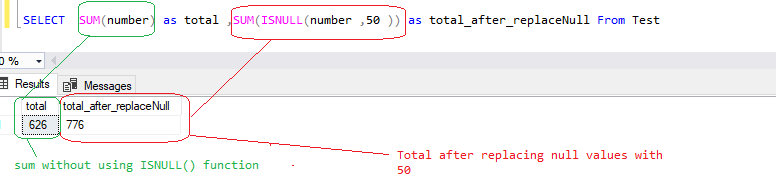Home » SQL SERVER ISNULL

# SQL SERVER ISNULLSQL Server ISNULL() function is a system function that returns the specified value if the expression is NULL else it returns the expression.

It returns the same type as expression.

#### SYNTAX

```ISNULL (expression, replacement_value )

```

expression
Is the expression to be checked for NULL. It can be of any type.

replacement_value
Is the expression to be returned if expression is NULL. It can be truncated, if replacement_value is longer than expression.

Lets look at an example of ISNULL() function in SQL Server.

Following statement uses ISNULL() and returns the specified value IF the expression is NULL, otherwise return the expression.

`SELECT ISNULL('microsoft sql server','2018') as output`As you can see , First argument that is an expression is not null so ISNULL() function returns first arguments value that is  ‘microsoft sql server’.

In case, if our expression (first argument) is null then it returns replacement value that means second argument values.

`SELECT ISNULL(null,'2018') as output`#### Using ISNULL() function with table data

Lets, create a sample table named as Test, then insert some records with null values.

```Create table test ( id int identity(1,1) ,number int )

insert into test values (12),(null) ,(14),(null) ,(null) ,(100),(500)````select * from test`As you can see, there are three null values in number column. We will use ISNULL() function to check if the value in number column is null then replace the null value with 1 else return value.

`SELECT number, ISNULL(number , 1 ) as number From Test`As you can see, it replaces the null values with 1.

#### Using ISNULL() function with Aggregated Function

Following statement uses ISNULL() function to replace the null values with 50 and then sum the values.

`SELECT SUM(number) as total ,SUM(ISNULL(number ,50 )) as total_after_replaceNull From Test`This site uses Akismet to reduce spam. Learn how your comment data is processed.